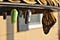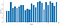# Automated feature engineering with evolutionary strategies.Photo by Suzanne D. Williams on Unsplash

Inside a data set there different samples of a population, each sample contains several features that show us how each sample is unique from any other sample. Those features can be used to train and evaluate a machine learning model. However, often those features are not able to make an accurate prediction, thus new features are needed to create a better and more accurate model. In this post, I will show you how to create new features from a dataset for a classification task.

# The data

The data consists of several features derived from audio files with two genres of music, pop music, and classical music. By counting the labels of each genre, we can see that the dataset is balanced.

Also, displaying the histogram of each feature in the data set we can observe that features like rmse, spectral bandwidth, and mfcc2, just to mention a few have what it looks like two distribution of values. That characteristic could be useful for the classification task.

Correlation among the different features show that tempo, beats mfcc18 and mfcc2 have the lowest correlation between the different features in the data set.

Now that we have a little bit of knowledge of the data set, we can begin to develop the evolutionary method for feature engineering

# The Feature strategy

As the data set contains only numerical features, we can apply a simple ratio as a feature skeleton, that function will help us to calculate the new feature.

As the ratio needs two features to be calculated, the evolutionary method will try to find the best combination of column indexes for the features to be calculated. For that, we create a function that generates a list of variable size lists filled with random numbers, with that strategy we can look also for a smaller model with a better performance. Then we change that index into pairs of values to calculate the new features.

With the index created, we calculate the new features from the index, then and process the new features to be used to train a random forest classifier. We train a min-max scaler from the skleran library and split the new data for training and testing, Finally, we train a random forest classifier from the sklearn library using the default parameters and calculate the ROC AUC as a measure of performance.

With all the functions in place to train the model and calculate the new features, we can apply the evolution strategies to optimize the model performance. In this example, we are going to use two strategies mutation and recombination. For the mutation strategy, we are going to use two different rules for mutation. The first one checks if the column index is of a determined size, if that its the case a random value in the column index will be deleted, otherwise a random element in the column index will be changed. That strategy will help us to reduce the model size. For the recombination strategy, a random column index will be selected from the population, then a random value from that column index will be inserted in another column index.

As the strategies are already defined, we can optimize the model, we train a population of column indexes and calculate the performance. Then we apply the evolution strategies to the initial population and calculate the performance. Finally, we update the population of column indexes only saving the ones with a better performance. Each time the evolution strategy is applied to the column index is called a generation.

By applying the evolutionary method to the classification task we can see that with only five generations we can find one model with high performance. And with twenty generations we can see that about one in five trained models has a high performance. Also by increasing the number of generations the average performance of the population also increases.Left five generations, right 20 generations, column width equals to model size.

Now you can define a basic workflow for automated feature engineering using evolutionary strategies. And some basic techniques for exploratory analysis. The complete data set used in this post can be found by clicking here, and the complete code can be found in my Github by clicking here. See you in the next one.

Writing about math, natural sciences and academia.

## More from Octavio Gonzalez-Lugo

Writing about math, natural sciences and academia.